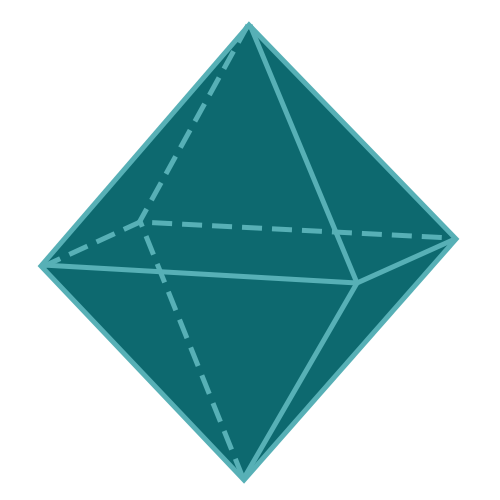Report a Problem
Suggestions

#Calculate The Volume of An Octahedron

Last updated: Saturday, June 24, 2023
Select a type of polyhedron below
Tetrahedron
Hexahedron
Octahedron
Dodecahedron
Icosahedron

An octahedron is a three-dimensional geometric figure with eight equilateral triangular faces. As one of the five Platonic solids, it has unique properties in geometry, making it relevant in various fields such as mathematics, engineering, and design. Studying octahedra enriches our understanding of the relationships between symmetry, shape, and structure.

Real-life examples of objects with an octahedron shape can be found in several contexts. In crystallography, octahedral structures are seen in the formation of minerals like diamond and fluorite, which possess strong interatomic bonds and a highly symmetrical arrangement of atoms. In art and design, octahedra are often used in sculptures and geometric decorations for their striking appearance and inherent balance.

In mathematics, the study of octahedra and other polyhedra helps deepen our understanding of three-dimensional geometry and its practical applications. The octahedron is the dual polyhedron of the cube, meaning it can be formed by connecting the centers of the faces of a cube. In engineering and computer graphics, octahedra can be used as building blocks for creating more complex shapes through a process called meshing, dividing irregular shapes into smaller elements for easier analysis and rendering.

Easily calculate the volume of an octahedron with step-by-step guidance using our free calculator below.

The formula for determining the volume of an octahedron is defined as:
$$V$$ $$=$$ $$\dfrac{\sqrt{2}}{3}$$ $$\cdot$$ $$a^3$$
$$V$$: the volume of the octahedron
$$a$$: the length of any side
The SI unit of volume is: $$cubic \text{ } meter\text{ }(m^3)$$

## Find $$V$$

Use this calculator to determine the volume of an octahedron when the length of any side is given.
Hold & Drag
CLOSE
the length of any side
$$a$$
$$meter$$
Bookmark this page or risk going on a digital treasure hunt again